# What is special about imaginary numbers

## Imaginary numbers

### - How science becomes mathematical intuition

Contribution to the topic of science and technology by 50plus active on Bergstrasse on November 22, 2017 in Bensheim, greatly expanded version, as of January 13, 2019

### introduction

Why are the imaginary numbers imaginary or impossible? What kind of imagination does they show? And why are imaginary numbers the insurmountable hurdle for most laypeople to understand the new content modern mathematics and natural science are concerned with? Is it possible to solve this with simple examples?

Are they just a helpful abbreviation so that electrical engineers and quantum physicists can calculate more easily, or are they an unbelievable jewel that the legendary Nobel Prize winner Richard Feynman raves about in his physics lectures?

Why are the biggest open questions in mathematics like the Riemann Hypothesis about the distribution of prime numbers here, and is it worth trying to understand topics of this kind at all?

Does the world consist of real or complex numbers, or possibly even exoteric numbers like the quaternions or the elements of the Lie group E8, which is composed of 248 complex dimensions?

For me, the imaginary numbers show Europe's new contribution to the history of mathematics: Just like the emergence of mathematics among the Egyptians and Sumerians under the heading How the pictures became numbers and the ancient mathematics of Pythagoras, Euclid and others bears the title How numbers became science, characterizes European mathematics How science became intuitive mathematics. While the natural numbers emerge from counting and the real numbers from measurement, the imaginary numbers are the result of mathematical imagination to construct mathematical relationships even in such areas and to gain verifiable knowledge with them, which are hidden from all sensory experiences and contradict the given rules of thought.

This should be shown in the development of imaginary numbers from the Italian polymath Cardano (1501-1576) to the present day, in the various areas of application of imaginary numbers and leads to the question of what new way of thinking is associated with imaginary numbers. Surprisingly, modern philosophy has hardly dealt with this topic so far, religion and theology certainly not, but writers like Robert Musil and psychologists like C.G. Jung and Lacan. This morning we shall try to shed some light on the mystery that surrounds the imaginary numbers.

### Complex numbers as a math game (Cardano)

The imaginary numbers go back to typical universal scholars of Renaissance humanism such as Girolamo Cardano (1501-1576), who emerged in various fields from medicine to philosophy to mathematics, as well as astrological interests and dream interpretation. Today his name is perhaps best known for the cardan shaft and cardanic suspension that are named after him. Renaissance thinkers like Cardano were thus on the one hand in the tradition of Arab scholars like Avicenna (980-1037) or Averroës (1126-1198) from the 10th to 12th centuries, who had a similar education and wealth of knowledge, but went in one crucial point beyond them: that is their mathematical intuition, which is what this post is about.

Cardano »has provided numerous horoscopes (including for Francesco Petrarca, Erasmus von Rotterdam and Albrecht Dürer) and has dealt with the interpretation of omens and premonitions. This earned him the reputation of a fanatic in the 18th century. Leibniz later judged him: 'It seems that knowledge has a magic that cannot be understood by those who have never been grasped by it. I don't mean just factual knowledge that has no reasons, but a knowledge like that of Cardano. He really was a great man, despite all his flaws; without them he would have been incomparable. ' (Leibniz: Essais de théodicée, 1710) «. (Wikipedia, accessed on August 26, 2017)

Its importance in the history of mathematics can be compared to that of the first mathematicians in Babylonia and the Pythagoreans. If they had introduced the first number systems and the geometric proof procedure, Cardano still stood 100 years before Descartes and Kepler for intuitive thinking or, more precisely, mathematical intuition, which became the paradigm for modern European mathematics, even if paradoxically it worked with it but never really realized it. Its innovation does not seem to me to have even come close to being appreciated. To this day, the question of imaginary numbers has remained almost unnoticed in Western philosophy.

His approach seems like a game - a thought experiment or a "glass bead game" (Hermann Hesse): He started from a task that is of no practical importance, but rather helps to follow previously unknown paths on a precisely posed question: There are two numbers a and b such that:

a + b = 10
a b = 40

All the main features of the imaginary numbers can be illustrated with this exercise. If you try different numbers, you will quickly notice that there is no solution in the area of ​​the usual numbers: For example, 1 + 9 = 10 applies to 1 and 9, but only 1 · 9 = 9. In the other extreme, 5 + 5 applies = 10, but 5 5 = 25 again does not reach 40.

A solution is only possible with complex numbers: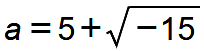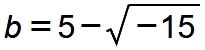Obviously a + b = 10 applies, since the square root of −15 is added once and then subtracted again. And if you do the math, you will find the product a b (after Gill, 1):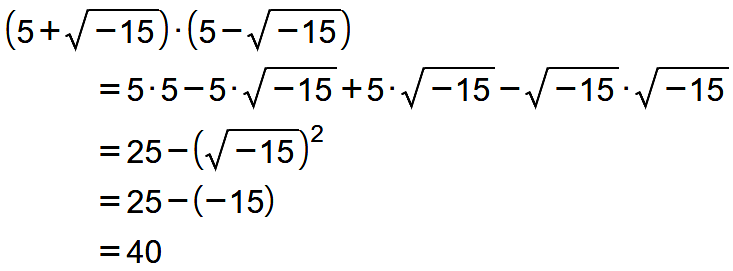What is the idea behind this solution? Cardano recognized that the solution cannot lie on the number line, but that the solutions must lie outside the number line. Under the chapter heading "De Regula falsum ponendi" (Rules of False Syntax) he spoke of an "impossible task" (quaestio est impossibilis) and of »quantitas sophistica«. It is not known how he found the solution. Various utterances suggest that he started from an algebraic idea when dealing with quadratic and cubic equations. He spoke of “dimissis incruciationibus”, which some translate as “overcoming mental torment” and others as “cross-wise products” and possibly contains a deliberately chosen language game. I want to try to illustrate it geometrically with a drawing and choose my own intuition, which will help to compare the different ways of multiplying with one another.Cardano's solution with imaginary numbers in my geometric representation

The drawing takes place in two steps:

- In order to find a solution for the problem a · b = 40, it can be assumed in the simplest case that a = b should apply. The number a is searched for, for which a · a = a2 = 40 should apply. That is the square root of 40. The square root of 40 is approximately 6.32. This number is entered on the real axis. That cannot be the solution yet, because 6.32 + 6.32 = 12.64 ≠ 10, so that the first condition is violated. At this point it is the intuition of mathematics not to limit itself to the number line, but to open a new dimension and make a circle around the zero point that intersects the real axis at 6.32. All points on the circle are at a distance of 6.32 from the zero point. The solution has to be somewhere on the circle, but not on the real number line.

- To find the points you are looking for on the circle, a straight line is drawn that is perpendicular to the real number line at the number 5. It cuts the circle with a radius of 6.32 at two points, and these two points of intersection are the solution we are looking for!

Cardano has not yet designated the imaginary numbers with the letter i, but he intuitively anticipated the calculation rules for imaginary numbers. The calculation rules for imaginary numbers must meet two requirements:

(i) You must not violate the known rules for natural and real numbers. If two real numbers are added or multiplied, the previously known results should remain valid.

(ii) If, on the other hand, two complex numbers are added or multiplied that do not lie on the real number line, then the calculation rules should be free of contradictions and should fulfill the task that Cardano had set himself.

The example of Cardano's task already shows how complex numbers are calculated:Addition and multiplication of imaginary numbers using the example of the Cardano problem

Addition: For the addition, the two distances that lead from the zero point to the respective complex number are carried out one after the other. In this example, the red line up to the number is firstgone, and then the blue route to the numberadded with a parallel shift (indicated by dotted blue), which results in the number 10 as desired. (Equally meaning, first the blue and then the red-dotted route can be carried out, whereby the 10 is also reached.)

Multiplication: There are two steps for multiplication:
(a) For every segment that leads to a complex number, the angle with respect to the real number axis is determined. In this example, the red line is rotated approximately at an angle of 50 ° with respect to the real number axis, the blue line at an angle of −50 °. When multiplying both diapers are added, which results in an angle of 0 ° in this example, since both numbers are mirror images above and below the real number axis. That was the solution idea based on Cardano's task: If the angles are added, the solution falls on the real number axis.
(b) At the same time, the lengths of the two factors are multiplied, that is, the distances between the two imaginary numbers and the zero point. In this example, that results in 6.32 * 6.32 = 40, since both numbers lie on the circle with a radius of 6.32.

It is not easy to understand the mathematical language in which Cardano found this solution. It was clear to him that there were no such numbers givesbut that they are exclusively the result of a mathematical imagination (phantasy, imagination). In an almost playful way, he anticipated the rules of complex numbers, which were only worked out in detail over the centuries after him. The representation that is still valid today was only introduced 300 years later by Bernhard Riemann. For me, Cardano's work is an outstanding example of what mathematical intuition is capable of.

### Rotation of complex numbers (cyclicity)

The calculation rules for imaginary numbers result very easily from the Cardano example. I am primarily concerned with the question: What is the new thing about complex numbers, which distinguishes imaginary numbers from ordinary numbers: The specialty of complex numbers lies in the multiplication of two complex numbers, in which the two factors are rotated. The easiest way to understand this is to interpret every complex number as a pointer that points from the zero point to the point of the complex number on the two-dimensional plane of numbers.

The Norwegian-Danish cartographer Caspar Wessel (1745-1818) and the Geneva-born bookseller, republican revolutionary and amateur mathematician Jean-Robert Argand (1768-1822) came up with this independently of each other around 1800. To this day, your approach cannot be more vividly formulated than in Wessel's words.

addition: “Two straight lines are added if we join them in such a way that the second line starts where the first ends, and then draw a straight line from the first to the last point of the joined lines. This line is the sum of the united lines ”(Wessel, § 1). This vividly generalizes the addition of ordinary numbers, in which the two pointers to the real numbers are placed next to each other on the number line. In the two-dimensional case, this results in a parallelogram, the diagonal of which is the sum sought. (The designation i for the imaginary axis goes back to Euler 1777. Complex numbers have only been spoken of since Gauss in 1831.)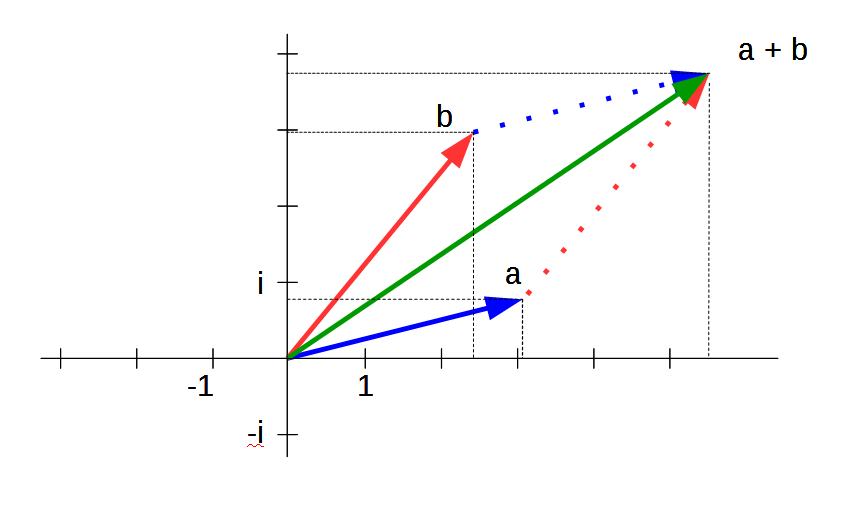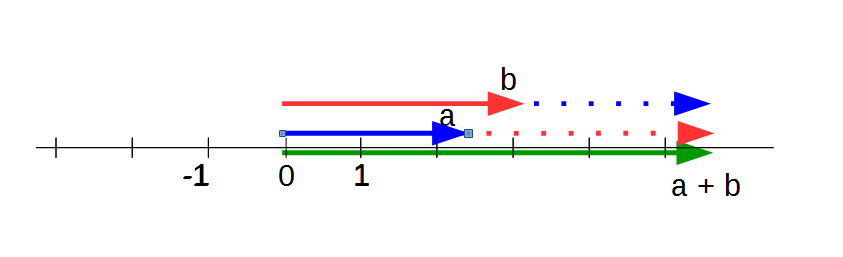Sum of two complex and two real numbers as a pointer

The complex numbers are represented with pointers (vectors): The red and blue vectors are added by adding their two coordinates. First the route of the red vector is taken, then the route of the blue vector, or equivalent: first the route of the blue vector, then the route of the red vector. A parallelogram is created. Its diagonal is the green vector. It is the sum of the two vectors a and b, or the equivalent of the two complex numbers a and b. The black dashed lines indicate the coordinates that are added together.

The same thing happens when real numbers are added, only in this case the red and blue vectors are on the same line. They are run one after the other. The result is the green vector as the sum. (Strictly speaking, the blue, red and green vectors lie on top of each other on the horizontal axis. In the drawing, they have to be shown on top of each other in order to be able to distinguish them.)

multiplication: In the words of Wessel, a "change of direction" (rotation) applies to the multiplication of two complex numbers. If two complex numbers are multiplied, this results in a new pointer, as with addition. Its length is the product of the lengths of the two factors. In addition, there is the “change of direction”, whereby the direction angle of the product “should be equal to the sum of the direction angles of the factors” (Wessel, § 4).Product of two complex numbers
Author: von Kmhkmh - own work, CC-BY 4.0, Link

The two complex factors are shown with a blue or red pointer, the product with a green pointer. The rotation results from the addition of the angles φ of the two pointers, the length as the product of the lengths of the blue and red pointers. In this example, the length of the blue pointer is greater than 1 and the length of the red pointer is less than 1. Therefore, their product gives a value that is between the lengths of the two factors. The product of two complex numbers results in a new complex number (green) through a twist extension.

As with addition, the rules for multiplying complex numbers are the same as the rules for multiplying real numbers: Positive real numbers can be viewed as complex numbers that are inclined at an angle of 0 ° (or 180 ° for the negative numbers). are rotated. If two real numbers are multiplied, the multiplication rules result directly from the position on the real number axis:

 Multiplication rule Adding the angles interpretation + · + = + 0° + 0° = 0° + · − = − 0° + 180° = 180° reflection − · + = − 180° + 0° = 180° Mirroring in the other direction − · − = + 180° + 180° = 360° = 0° full rotation to the starting point

The product of real numbers is again on the real number axis. It remains to multiply the amounts (the distances to the zero point) of the two real numbers and to take the signs into account, according to the usual rules.

In order to recognize how extraordinary a rotation (change of direction) is in mathematical operations, the idea of ​​the geometrical representation of Cardano's task should be examined one step more closely. It contains two moments: the scalar product and the vector product. The scalar product can be understood as a shadow image, the vector product with the establishment of a new axis and the product of complex numbers as a rotational extension that contains the scalar product and the vector product as internal moments. Anyone who learns mathematics, physics or technical subjects today is usually confronted with a variety of terms like these, and often in different contexts. Therefore, the impression can arise as if these are simply different possibilities with which the desired result is to be proven, which is already known in advance. However, starting with the multiplication of complex numbers, one can understand how these different types of multiplying internally belong together and what makes them different.

(i) Scalar product: It can clearly be said: Two pointers pointing in different directions cannot be multiplied directly with one another because they are twisted against one another. With the scalar product of two pointers, a way is found to take into account the rotation of both pointers to each other and to multiply it as if both were on the real number axis. In order to be able to multiply them, one pointer must be projected onto the other so that both lie on top of one another and point in the same direction. When that is done, they can be multiplied together like ordinary numbers. This is best seen in a simple drawing: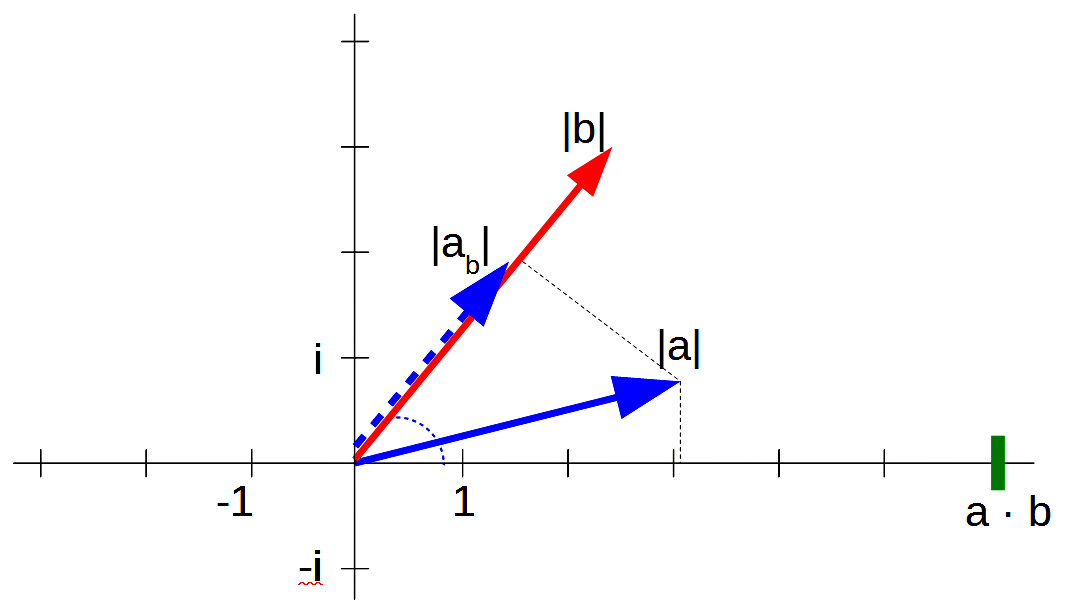Dot product

Left picture: The shorter pointer - in this example this is the blue pointer a with the length | a | - is projected onto the longer pointer.It is important to recognize the difference between a rotation and a projection. When rotating, the length does not change, when projecting it is shortened. The projection can be compared to the casting of a shadow: the projection is the shadow of one pointer on the other. This results in the blue dashed pointer ab with the length | ab | in this picture. You can see from the drawing that the length has become shorter with the projection: | from | is less than | a |. The scalar a · b highlighted in green is the product of the length of the longer vector, - denoted as | b | -, and the length of the shorter vector | ab | projected onto the longer vector. In this example this results in: a · b = | ab | · | B | .

If, in the limiting case, the angle between the two pointers is 0 ° and they lie on top of one another, then no projection is necessary and there is no need to distinguish between the length of the pointer before the projection - in this example, | a | - and the length of the projection - in this example this is | ab |. In this limiting case, the following applies directly: a · b = | a | · | B |.

Right picture: The idea of ​​the scalar product is already part of Cardano's intuition. If you look at the vertical line that starts from the real number 5, the shadows of the two pointers that point to the numbers are cast along this vertical lineandshow on the real number axis. While the two pointers each have a length of 6.32 (that is the radius of the circle), their shadow only has a length of 5. This can also be "read" in the other direction: Instead of the shadow being cast by the pointers on the real number axis "See", an extension can be seen conversely along the vertical, which extends from the pointer to the number 5 through a projection onto the two pointers to the numbersandleads. With this operation, the numbers are found that solve the problem: The product of their lengths results, as desired, 6.32 · 6.32 = 40, while their scalar product (that is the product of their shadows on the real number axis with the length 5 ) only gives 5 5 = 25. With this operation it was possible to "turn" 5 · 5 = 25

As an outlook it should be added that now only someone with a mathematical and physical imagination like Einstein had to come to transfer the game of mathematical intuition of numbers shown here to the movement space of mechanics and to recognize that the relationship between the The scalar product and the product of complex numbers occurring shortenings and extensions can be interpreted physically. This results in the transition to spaces in which there is length contraction in special relativity theory and curvature in general relativity theory. Curved space is related to flat space as the pointer rotated into the complex plane is related to its shadow cast on the real number axis. However, apparently neither Einstein nor Gödel noticed this connection. Working out this down to its philosophical consequences and finding, for example, elementary philosophical terms such as reflection, shadowing and imagination, their inner reference recognizable via the imaginary numbers, is the long-term goal of the work started with this article.

(ii) Vector product: Cardano's basic idea was undoubtedly to break away from the one-dimensional number line and open a new dimension in which the imaginary numbers lie. This basic idea becomes clearer when it is generalized to higher dimensions and is particularly illustrated at the transition from surfaces to space. In physics, this is provided by the three-finger rule, sometimes also referred to as the corkscrew rule or the right thumb rule. Here it is clearly shown how a new dimension standing vertically on them emerges from two dimensions.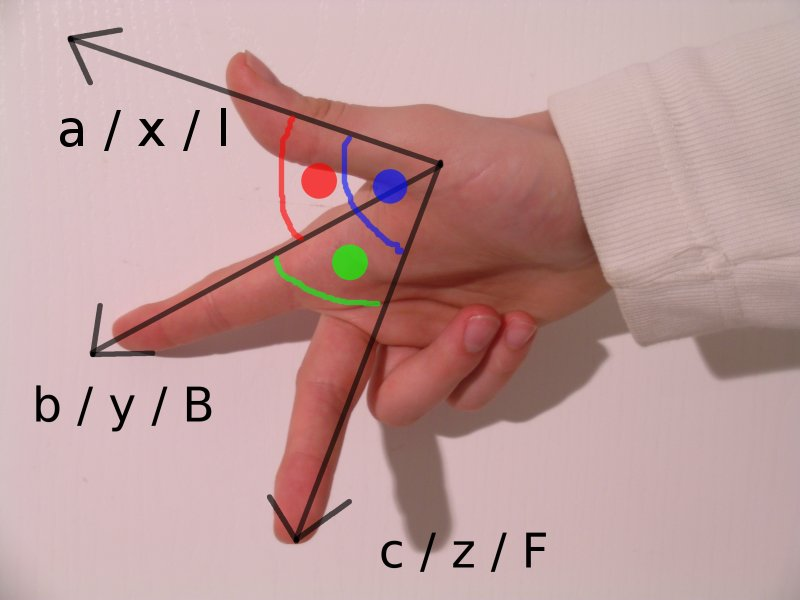Right thumb rule
The index finger and middle finger define an area. The thumb is perpendicular to the surface.
Author: Von Abdull - Eigenes Werk, CC BY-SA 3.0, Link

Electrical engineers use the rule of thumb to make it clear in which direction the current flows in a magnetic field. These are already examples of the applications of imaginary numbers in science and technology.

Mathematically, this is generalized to the vector product or cross product. While the scalar product gives a scalar - that is a real number - the vector product gives a vector. Two vectors that do not point in the same direction form a surface. The cross product of two vectors creates a vector that is perpendicular to this plane. (The cross product is sometimes misleadingly described as the outer product. The outer product is valid in outer algebras with its own calculation rules. This will be discussed in more detail in a later part.)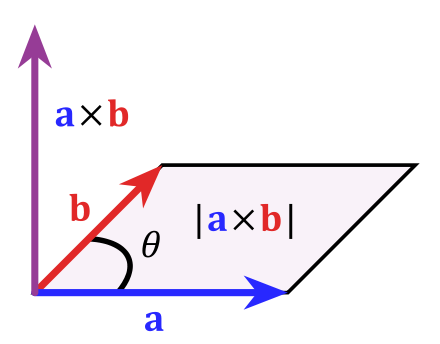Cross product
The cross product (vector product) of two vectors: Two vectors that do not point in the same direction form a plane. The vector product a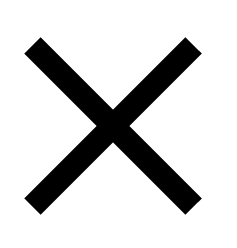b has a direction which is perpendicular to the plane formed by the two vectors. The numerical value of the vector product corresponds to the area of ​​the area formed by the two vectors.
Author: von MartinThoma - Eigenes Werk, CC BY 3.0, Link

Result This representation is intended to show two things: (i) The scalar product and the cross product can be understood as moments of the product of imaginary numbers. The scalar product generalizes the projection (the shadow cast) of two straight lines on top of one another, the cross product generalizes the opening up of a dimension and the rules that have to be followed. (ii) But neither the scalar product nor the cross product result in the rotation (the change in direction), which is typical for the imaginary numbers and distinguishes them from all other numbers. (Most of these findings go back to the Irish mathematician William Rowan Hamilton, 1805-1865. He not only deepened his understanding of complex numbers and, based on this, introduced other numbers such as quaternions, but also formulated vector arithmetic more clearly.)

The three types of multiplication are summarized side by side in order to see the similarities and differences:Product of two vectors and two complex numbers - overview
(a) The scalar product of two pointers (vector) results in a real number (highlighted in green)
(b) The vector product of two pointers (vectors) results in a pointer perpendicular to them (purple)
(c) The product of two complex numbers turns into a complex number (green)

Root of minus 1 After these preparations, the definition of imaginary numbers as the ‘Root of minus 1’ should be returned. The rotation makes it possible to find a number that, when multiplied by itself, results in a negative number. In the simplest casethe product i · i is considered: In this case, both factors (in each case the number i) have an angle of 90 ° relative to the real axis. If the two angles are added, this results in an angle of 180 ° and the product falls to the number −1 as a result of the rotation. Therefore i · i = −1 and after taking the roots we have i = on both sides.i · i as a rotation to −1
In this case, both factors (red and blue) are each the complex number i and an angle of 90 ° relative to the real axis. The product i · i = i2 = −1 results from adding the two angles to 180 ° = 90 ° + 90 °.

Hence it is misleading to say that the imaginary numbers are something like negative surfaces. They do not result from surfaces, but from rotation.

When complex numbers are multiplied together, it can cause the rotation to return to and exceed the starting point, like a clock. However, while the same numbers are run through every 12 hours on the clock, this should be avoided with the complex numbers. Every complex number should remain unique. This leads to a superposition of an infinite number of surfaces, which are arranged like in a spiral:Riemann area of ​​the complex logarithm
Author: Von Leonid 2 - Own work, CC BY-SA 3.0, Link

With this representation, the special thing about complex numbers is met. The complex numbers are characterized by a multiplication in which two complex numbers are rotated, and the rotation can spiral into continuously new leaves. The image of a screw that flows from bottom to top is vividly created, or in the language of the Far East of a snake that ascends through a series of wheels and gains new energy and changes with each wheel. Complex numbers are therefore particularly suitable for the mathematical description of processes that both rotate and flow.

### Fluid mechanics according to Euler

For Cardano, the complex numbers were still a kind of gimmick and a challenge for mathematical intuition, but 200 years later Leonhard Euler (1707-1783) made the transition to diverse areas of application in mathematics and physics. In mechanics he no longer assumes rigid bodies and in mathematics no longer assumes dimensionless points, but rather flows and their mathematical shape in at least two dimensions. In a current there is constant turbulence and an inner movement. The flow can flow in one flow direction as well as rotate around its own flow axis (which has to be taken into account especially with electrical currents).

The flow behavior should be illustrated using a current example:Streamlines around a car
Author: Von AndreHuppertz, CC BY-SA 3.0, Link

The air flow is not punctiform, but clearly referred to as a flow tube and inside of a flow thread, which can be reproduced and simulated with wind tunnels. Turbulence forms on the back of the car that impair driving behavior.

Euler dealt with numerous examples of this type of application. From today's perspective, he anticipated the paradigm shift in mathematics in the 20th century to differential geometry and the theory of differentiable manifolds. He himself was a practitioner who concentrated entirely on solving a large number of specific tasks. He has not yet developed a theoretical framework for this, but has introduced a large number of symbols that are still used today, with which he simplified his arithmetic, including symbols, i and e for the circular functions, circulating movements and the imaginary numbers (Euler's formula). His pragmatic approach is probably the explanation why he is recognized as an extremely productive mathematician, but the scope of his new founding of mathematics and mechanics as fluid mechanics remains unrecognized. Hegel refers to Euler only via Lagrange with regard to the differential calculus (HW 5.303-306), but nowhere goes into the complex numbers and the new ideas of Euler. Basically, as was the case with Euler, imaginary numbers are still predominantly seen as a kind of technical aid and not as a completely new way of thinking in mathematics (cyclical thinking).

### Pointer model: complex numbers in science and technology since 1900

Nothing about that has changed with the next major area of ​​application, electrical engineering. On the contrary, it has cemented the reputation of imaginary numbers as a tool for engineers and technicians. The first technical inventions date back to around 1880 and have since completely turned the economy and our lives up to the point of everyday life. With electrical engineering and electrification, electrical currents were used, from power distribution via electric motors to the use of radio devices. In all cases it became necessary to precisely determine and regulate the magnitude and influencing factors of the currents, and in most cases calculating with complex numbers is easiest. This is where the rule of thumb arose.

The basic idea is the pointer model, with which Euler's formula for circular functions is expanded. Periodic oscillations of electrical circuits, for example, are interpreted as the timing of a pointer that rotates like a clock hand in a circle. If the pointer is understood as the rotation in the complex plane, then the chronologically periodic processes can be written down comparatively easily as a complex function.Pointer model
»The vector diagram (left) using the example of a spring pendulum: In the diagram (right) the instantaneous deflection y (t) of the pendulum is plotted against time. As you can see, it corresponds to the projection of the pointer onto the y-axis. "(Accessed on August 27, 2017)
Author: From Pyrrhocorax in Wikipedia in German - Own work of the original uploader, public domain, link

The complex alternating current bill was first introduced in 1893 and summarized in 1937. In an electrical oscillating circuit "energy is periodically exchanged between the magnetic field of the coil and the electrical field of the capacitor, which means that high currents and high voltages alternate" (Wikipedia entry, accessed on August 27, 2017). The easiest way to describe the cyclical process is with complex numbers. The applications range across electrical engineering.

### Quantum theory

Only with quantum theory does the attitude towards imaginary numbers begin to change. The basic idea has remained the same if the atom, like an electrical circuit, is understood as a vibrating system that can best be described with complex numbers. But anyone who sees formulas like the Schrödinger equation for the first time, in which the imaginary unit i occurs, has elementary difficulties imagining what and how that is meant. Two things come together here: firstly, hardly anyone understands why quantum physics only knows probabilities, and secondly it is difficult to imagine how probabilities change periodically and can therefore be formulated most easily with imaginary numbers.

(i) It begins with the question of quantum physics, where a particle can be detected and measured, its probability of being. In the case of a freely swinging pendulum, under ideal conditions it can be calculated exactly where the pendulum is. According to the pointer model, there is a sine function that describes how the pendulum swings up and down. In today's quantum theory, it is no longer possible to describe exactly where a single particle will be located (alternative models such as that by David Bohm are predominantly rejected, see the article on basic questions in quantum physics). But it is possible to calculate the probability of where it can be detected. However, the probability of the location is not always the same, but changes periodically like the movement of a pendulum and is therefore described with imaginary numbers in accordance with the pointer model. This, too, must first simply be accepted as an empirically confirmed fact.Probability of stay
»Wave function (in red), probability density (blue) and location probability (green) of the second excited state (n = 2) of a one-dimensional harmonic oscillator.
The probability of finding a particle in the interval A (green area: -2 Author: Von Debenben - Own work, CC BY-SA 3.0, Link

The probability of stay depends on the respective time interval. It is shown in this diagram as a green area that applies to the time interval under consideration of the periodically changing probability density (blue curve).

(ii) It becomes even more complicated if not only the location but the state of a particle in quantum physics is considered. The state can only assume a finite number of possible values, and only the transition probability with which the particle jumps from one state to another can be calculated. The transition probability in turn changes periodically and is therefore represented with circular functions like the residence probability.

### Dynamic color gradients

Despite all experience in the applications, the special properties of complex numbers are difficult to understand because it requires a great deal of rethinking to be able to imagine how a function of complex variables works. Everyone knows the graphs (value progressions) of real functions, be it the trajectory parabola, in which place and time are the two coordinates of a movement, or representations of economic changes over time, e.g. if the development of the gross domestic product of a country is shown over time. In the case of real functions, a point-shaped, variable value x is assigned another point-shaped value y = f (x), and both result in the points (x, y) of the motion curve. But how should it be possible to imagine a function in which the punctiform x is replaced by a complex number z with 2 coordinates (its real and imaginary part), and also the function value f (z) has two coordinates?

Dynamic color gradients are the standard method for this today.Their basic idea is to assign a color value to all points on a surface, which are each defined by their x and y coordinates, which also has two coordinates: the hue and the brightness. The changes in color gradients can be shown with the aid of animations. This can be understood as the course of a complex function with which a color value (hue, brightness) is assigned to each point (x, y). This is used in imaging processes and has now become one of the most important areas of application for imaginary numbers, which are used not only in medicine, but by almost all sciences. Anyone who can "read" the representation of color gradients understands the course of a complex function. One variant are the fractals, with which color values ​​are also assigned to all points of a two-dimensional figure.

There are precursors that help make complex functions easier to understand. The oldest example are the topographic maps introduced in the 18th century. Any place (topos), like complex numbers, has two coordinates (the degree of elevation and degree of latitude). The respective height is entered with the color, and sometimes the respective gradient with an indicated shadow (hillshade). The topographic map with hillshade is an example of a complex function: A number with two coordinates (longitude, latitude) is mapped to a value with also two coordinates (height as color, slope as hillshade). Mathematically, it is the representation of a four-dimensional space (longitude, latitude, altitude, slope) on a two-dimensional surface (the map sheet).Map with and without shading
“Lake Mead, USA
Above: map without shading;
Below: Map with oblique light shading (screenshot from NASA World Wind Software) «(Wikipedia, accessed on November 22, 2017)
Author: Gemeinfrei, Link

The Chladnian sound figures known since 1787 are even more impressive. A violin string is applied to a plate sprinkled with thin sand and begins to vibrate. The grains of sand are arranged in symmetrical patterns. At every single point you can see in which direction a grain of sand is arranged and how close the pattern is at this point. Therefore, like the topographic maps, it is another example of a complex function: Each location on the vibrating surface is assigned a value with two coordinates: the direction of the grain of sand and the density of the grains of sand at this point. The direction of the grains of sand is represented by the direction of the lines, the density by the size of the empty areas between the lines.Chladnian sound figures
Author: Von E.F.F.Chladni - From the book "Die Akustik" from E.F.F.Chladni. Originally from de.wikipedia; description page is / was here., Public domain, Link

Figures of this kind were known to Hegel, Schopenhauer and Nietzsche. In his natural philosophy on the concept of tones, Hegel mentions the "nodes of oscillation" according to Chladni (Hegel Enz. § 301, HW 9.176) and has in the Science of logic possibly developed the concept of nodal lines based on their model (HW 5.435). Schopenhauer calls in The world as will and idea Section 52 the Acoustics from Chladni (in which the sound figures are dealt with) when he speaks of the harmonic systems and the dissonances of the tones. Nietzsche speaks in About truth and lies in a non-moral sense (1873) of a deaf person who "marvels at the chladnian sound figures in the sand, finds their causes in the trembling of the string and will now swear by it that he must now know what people call the 'tone'". In this way they approached a phenomenon that leads to the question of imaginary numbers, but for them it apparently remained nothing but one of the many curious examples with which the romanticism of nature liked to deal. They did not yet recognize the hidden properties of the complex numbers and their functions, the cyclicity, which goes far beyond these examples in importance and requires a renewed philosophy of mathematics.

Engineers and technicians preferred a different representation that is already closer to a mathematical representation. You enter arrows on a surface, whereby each arrow is defined by its direction and size (length) and at the same time the density of the arrows can be varied.Function graph ofIn the representation, the image of the complex function is drawn in at the location of each complex number. Its real part is the length of the arrow, its imaginary part is the direction of the arrow. According to the function, two poles can be identified. This can be interpreted as the description of an electric or a magnetic field.
Source: Wikibook, accessed on November 21, 2017

Numerous other examples can be found on the nascent Wikibook on Complex Numbers. There, there is a step-by-step transition from the representation of real to complex functions and the complexity of the functions is gradually increased.

Only with the free use of color and the technical possibilities of computer graphics do the richness and application possibilities of complex functions become clear. In these examples, a color value with the two coordinates hue and lightness is assigned to each location.Function graph ofIn the representation, the image of the complex function is drawn in at the location of each complex number. Its real part is color, its imaginary part is brightness.
Source: Wikibook, accessed on November 21, 2017

With animations, it is also possible to illustrate complex numbers over time. There is either a change in the color gradient or a movement of the surface on which the colors and their brightnesses are entered. A nice example is the complex tangential function when its real and imaginary parts are changed over time:Function graph of tan z
Source: Wikibook, accessed on November 21, 2017

Due to their cyclical nature, the complex numbers are of particular importance for fractals. If numbers are chosen that cause spiral-like developments, self-similarity can easily arise.

»The Mandelbrot set is the set of all complex numbers c for which the through
z0 = 0
zn + 1 = zn2
recursively defined sequence is bounded. Images of the Mandelbrot set can be generated by assigning a color value in the complex plane for each value of the parameter c, which remains finite according to the above recursion. «(Wikipedia entry on the Mandelbrot set, accessed on August 27, 2017)Amount of almond bread
Author: The authorship was not given in a machine-readable form. Wolfgangbeyer is assumed to be the author (based on the information provided by the rights holder). - The authorship was not given in a machine-readable form. It is assumed that this is a separate work (based on the information provided by the rights holder)., CC BY-SA 3.0, Link

Film sequences that zoom in on amounts of Mandelbrot are particularly impressive. See a selection on YouTube with the search term "mandelbrot fractal zoom".

### Cyclical thinking

Despite all the applications and the significantly improved possibilities of graphic representation, the imaginary numbers are so unusual to this day that not only laypeople have difficulties with them, but philosophy, psychology or theology have largely ignored or can not do anything with them. Anyone who deals with this topic is breaking new ground. I would like to propose the thesis that imaginary numbers are associated with their own way of thinking, which is no less important than causality and principle thinking, which are based on natural or real numbers. It is not just a matter of using complex numbers to optimally formulate the course of turbulence or other gyrating movements, but also to understand cyclicity as a property of thinking itself: Just as the complex numbers move away from the real area until If there is a reversal in the imaginary area that finds its way back to the real numbers, cyclical thinking succeeds in turning away from the close connection to the real (the facts) into a field of possibility, and there a reversal back into the thinking of the real. Just as this is possible for imaginary numbers through a clear rule of multiplication, a new logic has to be designed for cyclical thinking, which is groundbreaking Laws of Form (Laws of Form) by Spencer-Brown. For me, comments on his work are the first step in thinking about cyclicality.

At this point, only the few exceptions with which complex numbers have been discussed in literature, philosophy and psychology will initially be presented. The own ideas on cyclical thinking that build on this and lead further are to be elaborated in further contributions. So far:

- A commentary on Spencer-Brown's logic, which speaks of imaginary numbers in a completely new way.

- A contribution about the cyclicality of imaginary numbers at the 41st International Wittgenstein Symposium in Kirchberg am Wechsel on August 10th, 2018

- A post about dynamic, dreamed and imaginary numbers from 2018

Further contributions are planned on cyclical thinking according to Raymond Swing, on questions of modern natural science about Buddhism, which also concern cyclical thinking, and on the expansion of this approach.

### Robert Musil on imaginary numbers in The confusions of the pupil Törless (1906)

Before philosophy, literature became aware of imaginary numbers. Even philosophers like Hegel, Schopenhauer, Frege or Husserl, who had studied mathematics intensively, simply overlooked them, and even Russell and Gödel did not mention them. But when in the 19th century, contrary to the materialistic zeitgeist, there was a turn from realism to unrealism and literature began to become aware of its independent position with symbolism, Robert Musil (1880-1942) left both in his first novel, published in 1906 The confusions of the pupil Törless meet directly. Unlike most of the writers and philosophers of his time, Musil had completed training at a technical university and passed the second engineering examination in 1901 with the overall grade of "very capable". He sensed that the imaginary numbers were creating something new that went far beyond mere arithmetic conventions, but received no answer. in the Törless he puts this question in the mouth of his fictional character.

Törless has a vague expectation about "the terrible things" that happen in mathematics. As soon as he entered his teacher's room and saw its simple furnishings, "his respect [...] began to give way to suspicious reluctance" - not only for the teacher, but also for mathematics. The teacher senses the question of the transcendent and supernatural and fends off it. He answers what everyone has probably heard like this or similar:

“So, as far as mathematics is concerned, it is quite certain that there is also a natural and only mathematical connection here. Only, in order to be strictly scientific, I would have to make preconditions which you should hardly understand, and we don't have the time to do so. "
“I like to admit that, for example, these imaginary, these non-existent numerical values, ha ha, are no small nut to crack for a young student. You have to be content with the fact that such mathematical concepts are purely mathematical necessities of thinking. "

The teacher sees his students "drawn away from science to religious viewpoints" and recognizes in them "a sensitive mind for the finer, I should like to say divine and transcendent nature of morality", to which mathematics has no answer for him , not even with the imaginary numbers.

Musil's novel, however, remained an exception in literature. It was not until 100 years later that the American writer Thomas Pynchon (* 1937) took up the subject again. Pynchon had first studied physics and after the war worked as a technical editor for the aviation company Boeing. In his great novel Against the day (2006) he writes in detailed accounts of the enthusiasm for complex numbers, quaternions and the Riemann Hypothesis, which in 1900 had captured a whole generation of scientists and, not least in Oxford and Göttingen, created an almost magical atmosphere from which both the physical views of the Relativity and quantum theory as well as the new philosophical current of phenomenology emerged.

### Cassirer on the Imaginary (1910 and ca.1930)

For Ernst Cassirer (1874-1945), who was 6 years older than him, as for most of the philosophers of his time, Kant was the great model, but he soon turned from Kant to Leibniz, who had almost been forgotten by Kant. With Leibniz, Cassirer gained a new perspective on mathematics and did so in his early major work Concept of substance and concept of function from 1910 saw a turning point in mathematics from operating with numbers to operating with symbols using the example of the symbols introduced by Leibniz for the differential calculus. Natural numbers relate directly to natural objects (substances) and their numbers, while mathematical symbols are used to consider relations between mathematical objects that only exist in thinking and the imagination of mathematics.

Wouldn't it have been obvious to take a closer look at the symbols for imaginary numbers introduced by Euler? However, only a few remarks about imaginary numbers are known from Leibniz. He calculated with them and found astounding results, they in 1702 as a “fine and wonderful refuge of the divine spirit, almost a hybrid between being and non-being” (“taque elegans et mirabile effugium reperit in illo analyzeos miraculo, idealis mondi monstro, pene inter Ens et non Ens amphibium quo radicem imaginariam apellamus «) (Wikipedia accessed on November 17, 2017, German translation by Remmert, 48), and on the whole he had a high opinion of Cardano.

Cassirer, however, did not see a new way of thinking in mathematics and perhaps even human reason as a whole in imaginary numbers, but for him a development is completed with imaginary numbers that has already led to the introduction of negative, irrational and transfinite numbers. With reference to Gauss, he differentiates between numbers that have a substance character and numbers with a relational character. On the one hand, there are tangible objects with their own substance that can be counted. Anyone who speaks of numbers like 2 or 4 can easily imagine objects with a number of 2 or 4. It is different with the negative numbers. There are no items with a negative number. The negative numbers arise only in the thinking and in the imagination of mathematicians when they imagine numbers in a purely formal way that are opposite to the known positive numbers. Cassirer quotes Gauss:

“Positive and negative numbers can only find an application where what has been counted has an opposite, which, combined with it, is equal to annihilation. On closer inspection, this presupposition only takes place where it is not substances (objects that are conceivable in themselves) but rather relations between two objects that count. "(Cassirer, 72 with a quotation from an advertisement by Gauss about the metaphysics of imaginary numbers from 1831 ).

This is repeated for Cassirer with the irrational numbers, the transfinite and finally the imaginary numbers. For example, if you look at the diagonal in a square, you can see an object with the number 1 in it, "a diagonal". But their length does not result from their object character, but rather as the relation of the diagonal to the outside of the square, as it was drawn according to the geometric construction. Only in the geometric construction do irrational numbers appear in the area of ​​relations, which differ fundamentally from simple numbers of tangible objects (substances). Cantor constructed the transfinite numbers in a similar manner, and this is how Cassirer understands the imaginary numbers. They do not result from the consideration of real objects, but from a mathematical construction with a real and an imaginary axis, which only exist in mathematical thinking.

“The concept and the designation of the 'imaginary' number is the expression of a thought which, according to its first approach, is already effective in each of the new types of number and which gives it its characteristic stamp. They are judgments and statements about the 'non-real', which nevertheless claim a certain, indispensable cognitive value for themselves. "(Cassirer, 71)

With this, Cassirer found a basic property of imaginary numbers, but deliberately does not distinguish the peculiarities of imaginary numbers from irrational numbers, for example. For him, all these numbers have arisen from mathematical thinking and describe relationships.In addition, the question of the imaginary took a back seat for him. The preparatory work for a planned 4th volume for the Philosophy of symbolic forms known from which Philipp Stoellger (* 1967) in his contribution The imaginary of the philosophy of symbolic forms with the subtitle To the imaginary as a figure of the third between the symbolic and the real quoted. There Cassirer goes even further: he sees the imaginary numbers not only in common with the negative, irrational and transfinite numbers, but also with the culture as a whole.

»[T] he culture [...] is never real [,] but one complex Size (a + bi) [,] because it contains an ›imaginary‹ (meaning) unit [.] «(Cassirer Postponed manuscripts and texts Vol. 1 On the metaphysics of symbolic forms, Hamburg 1995, 245, quoted from Stoellger, 393).

According to Stoellger, for Cassirer the imaginary numbers are the model for the special status of every culture far beyond mathematics. Culture is anchored in real life and emerges from it, can detach itself from it and create its own realm of imagination, which however does not simply float in nothing or is separated from real life by a magical border, but with it remains connected and leads back into it. According to Stoellger, with Cassirer »the imaginary as the figure of the third between the symbolic and the real to understand. The fact that in the symbol of the 'imaginary' religion and art can also be understood differently than just the relationship between the symbolic and the real “is a consequence” (Stoellger, 395). What Cassirer means by culture that moves away from real life and returns to it can roughly be compared to the idea of ​​cyclical thinking, but sets the focus differently and moves away from the concern of finding its own logic for it.

Philosophy has remained at this level ever since. A generation after Cassirer, the American philosopher William Van Orman Quine (1908-2000) was looking for an answer of his own. For him, the imaginary numbers are an example of holism. In themselves they seem impossible, and their meaning and use only emerge in the whole of the system. This is already indicated by Cassirer when he speaks of the totality of the real, the symbolic and the imaginary. In 2014, Michael Otte gave tips on the philosophical discussion of imaginary numbers.

“From the 17th to the 19th centuries, the imaginary numbers even remained mere mathematical marks, until Gauss, among others, suggested a geometric modeling for them, and this model in turn gained its confirmation from internal coherence and external application. It is very often the meaning and not the meaning that is important and initially helps! "(Otte, 117)
"In the algebra Cardanos (1545) and Bombellis (1572) 'imaginary' numbers are used for calculations, the meaning (sense) of which lies purely in their use and which, as their name suggests, do not represent anything empirically large." (Otte, 165f )

### Lacan on Imaginary Numbers (1958-1962)

Psychology, too, reacted surprisingly late to imaginary numbers, although questions of the imagination and, in the narrower sense, of the imago are among the basic concepts of psychology. Sigmund Freud (1856-1939) made a paradigm shift in the field of dream interpretation and made dream interpretation accessible to scientific research for the first time by trying to prove his own language or dream logic in dreams (compression and shifting of dream contents), but he did never seems to have seen a possible connection to the imaginary numbers. Carl Gustav Jung (1875-1961) worked through Imago and had known the physicist Wolfgang Pauli (1900-1958) since 1931. Pauli came to him for psychological treatment. The cyclical nature of quantum mechanics followed him into his dreams. In a letter to Jung, he reports the following dream:

»Dream: (...) Then the blonde speaks: 'The men whose wives objectified the rotation are accused.' (...) Soon after the negro left with the note, my wife really comes and says to me: 'You forgot to say good night to me'. (...) With the haunted tone of a teacher he (the blonde, t.) Continues: 'But you you know what rotation is! ' 'Of course' I immediately say 'the cycle and the circulation of light that is one of the initial reasons'. (Then the blonde says: 'Now you understand the men whose wives have objectified the rotation for them.' Now I kiss my wife and say to her: 'Good night! It is absolutely terrible what these poor people who are accused are suffering I get very sad and cry. But the blonde says with a smile: 'Now you hold the first key in your hand. "(Letter from Pauli to Jung on October 28, 46, quoted in Pauli, 34f)

There was also a scientific collaboration. In a joint brochure Pauli published a work on Kepler and Jung on synchronicity. But the topic of imaginary numbers was not taken up. This only happened with Lacan, with surprisingly similar results as with Cassirer.

Jacques Lacan (1901-1981) developed a concept of the imaginary which went far beyond mathematics and which, for him, determined human existence and thinking as a whole. For him, the real, the symbolic and the imaginary are three areas that depend on each other and can only be explained alternately with one another. Seen in this way, Lacan consistently continues Quine's holism in structuralism, but he does not limit his investigation to the position of imaginary numbers within mathematical calculations. But what he means can be shown more clearly with the imaginary numbers than with other examples: The imaginary numbers would remain mere dream structures if they were not created according to clear rules on the basis of real numbers and returned to them. These rules can only be formulated if a specific symbol language is found. On the other hand, the real numbers (the real) would remain flat and dead, were it not for the imagination with which the motion of the imaginary numbers emerging from them can be described. And so would the symbols like e, i, or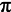to remain meaningless if it were not for the movement and operations of the imaginary numbers with which and on which they are carried out.

Rolf Nemitz compiles the passages in which Lacan speaks of imaginary numbers:

“An imaginary number is a number whose square is a negative real number, for example. Instead of 'imaginary numbers', people used to speak of 'impossible numbers' (cf. Lacan: 'The real is the impossible').
Lacan refers to imaginary numbers for the first time in seminar 6, Desire and its interpretation, 1958/59 (meeting of April 22, 1959), he says here that human life can be defined as a calculus whose zero is irrational, and he adds that this is a mathematical metaphor and that the term 'irrational' relates to an imaginary number,. He then explains that by this he understands a signifier that cannot be subjectified. (See Seminar 6, Miller version p. 387 f.)
In the essay Subversion of the subject and dialectic of desire in the Freudian unconscious (written in 1962) it says about: 'It is what the subject lacks to be through Cogito to think exhausted, namely what it is of the unthinkable ', an' absence in the sea of ​​proper names' (J. Lacan: Fonts. Volume II. Complete Text. Translated by Hans-Dieter Gondek. Turia and Kant, Vienna 2015, pp. 325-368, here: p. 358). This will be explained in more detail in seminar 9, The identification, 1961/62, at the meetings of January 10, 1962 and April 2, 1962.
The expression 'function of the real numbers' stands for the function by which - as Lacan assumes - the real numbers can be defined. «(Nemitz Imaginary numbers with Jacques Lacan, Footnote 36)

Alain Sokal (* 1955) criticized Lacan in Seminar 6 equates irrational and imaginary numbers and apparently "did not understand the matter on which he based his assertions" (Marius Erdt Elegant nonsense, Koblenz 2004, 13). From Cassirer's perspective, however, it becomes clear what Lacan meant by that.

In terms of content, Lacan was able to fill Cassirer's basic idea with many examples from psychology and found impressive images such as the Borromean knot to illustrate the interdependence of the real, the imaginary and the symbolic, but he did not go deeper into the mathematical properties of imaginary numbers either .

### literature

Hans-Jürgen Caspar: Imaginary circle, link

Ernst Cassirer: Concept of Substance and Concept of Function, Berlin 1910

Richard Feynman: The Feynman Lectures on Physics, Volume 1, Chapter 22: Algebra; link

Wilhelm Forst and Dieter Hoffmann: Exploring Function Theory with Maple; link

Klaus Fritzsche: Basic Course Function Theory, Heidelberg 2009
Excerpts and bonus material on the Springer-Verlag website

Lutz Führer: Cubic Equations and the Reluctant Discovery of Complex Numbers; link

Helmut Gericke: Mathematik im Abendland, Berlin et al. 1990

Brian Gill: Why i? The Historical Roots of 'Imaginary' Numbers, Link

Thomas and Brigitte Görnitz: The creative cosmos, Heidelberg, Berlin 2006

Hans Humenberger: How did the complex numbers get into mathematics ?; link

Jacques Lacan: Seminar XVIII, session 8 (May 19, 1971); link

Christian B. Lang, Norbert Pucker: Mathematical Methods in Physics, Heidelberg 2010 

Maths planet: existential function theory - Sartre's residual theorem; link

Rolf Nemitz: Imaginary numbers with Jacques Lacan; lacan_entziffer from January 27th, 2017

Max Neunhöffer: How did E8 make it into the headlines ?; link

Michael Otte: Analytical Philosophy, Hamburg 2014

Wolfgang Pauli, C.G. Jung: A correspondence 1932-1958, Heidelberg et al. 1992

Helmut Pulte: The principle of the smallest effect and the force conceptions of rational mechanics, Stuttgart 1989

Reinhold Remmert: Complex numbers
in: Ebbinghaus et al. (ed.) numbers, Berlin, Heidelberg 1983

Jürgen Roth: The number i - fantastic, practical, clear
in: Mathematik lehren, Issue 121, December 2003, pp. 47-49; link

George Spencer-Brown: Laws of Form, New York 1972 (Julian Press) ; link

Philipp Stoellger: The imaginary of the philosophy of symbolic forms
in: Birgit Recki (Hg), Philosophy of Culture - Culture of Philosophizing, Hamburg 2012, 393-420; link

Raymond Swing: Formal and Generative Dialectics, Copenhagen 2006; on-line

Caspar Wessel: On the analytical representation of direction, ; link

Wikibooks: Complex Numbers: Representation of complex-valued functions - math for non-freaks; link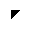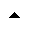& copy tydecks.info 2017